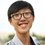# Chemistry - Boyle's Law

As mentioned in my previous post, the studies of gases are much related on Volume, Temperature and Pressure.

For Boyle's Law, it studies the relationship between Volume and Pressure of a gas.

Definition: The pressure of an ideal gas at constant temperature varies inversely with its volume.

By mathematical expression, it will be:

$V\propto\frac{1}{p}$

where

$V$ is for volume

$p$ is for pressure

When the pressure increase, the volume decrease;

When the pressure decrease, the volume increase.

If you squeeze a balloon, you increase the pressure, then you will see the balloon contracted.

If you let your balloon floats up to the sky, the air pressure decrease and your balloon will expand.

So, as the temperature is fixed, we will have:

$p_1V_1=p_2V_2=k$

where $k$ is the proportional constant

Some other notes:

1. For Boyle's Law, the mass of the gas must be fixed.

2. For Boyle's Law, the temperature is fixed.

3. Boyle's Law is only suitable when the gas we are dealing is an Ideal Gas.Note by Christopher Boo
6 years, 7 months ago

This discussion board is a place to discuss our Daily Challenges and the math and science related to those challenges. Explanations are more than just a solution — they should explain the steps and thinking strategies that you used to obtain the solution. Comments should further the discussion of math and science.

When posting on Brilliant:

• Use the emojis to react to an explanation, whether you're congratulating a job well done , or just really confused .
• Ask specific questions about the challenge or the steps in somebody's explanation. Well-posed questions can add a lot to the discussion, but posting "I don't understand!" doesn't help anyone.
• Try to contribute something new to the discussion, whether it is an extension, generalization or other idea related to the challenge.

MarkdownAppears as
*italics* or _italics_ italics
**bold** or __bold__ bold
- bulleted- list
• bulleted
• list
1. numbered2. list
1. numbered
2. list
Note: you must add a full line of space before and after lists for them to show up correctly
paragraph 1paragraph 2

paragraph 1

paragraph 2

[example link](https://brilliant.org)example link
> This is a quote
This is a quote
    # I indented these lines
# 4 spaces, and now they show
# up as a code block.

print "hello world"
# I indented these lines
# 4 spaces, and now they show
# up as a code block.

print "hello world"
MathAppears as
Remember to wrap math in $$ ... $$ or $ ... $ to ensure proper formatting.
2 \times 3 $2 \times 3$
2^{34} $2^{34}$
a_{i-1} $a_{i-1}$
\frac{2}{3} $\frac{2}{3}$
\sqrt{2} $\sqrt{2}$
\sum_{i=1}^3 $\sum_{i=1}^3$
\sin \theta $\sin \theta$
\boxed{123} $\boxed{123}$

Sort by:

Thanks for all your posts, by the way, what is an ideal gas??.

- 6 years, 4 months ago

Very good

- 6 years, 6 months ago

Awesome job! (y)

- 6 years, 7 months ago

Nice Post!

Waiting for notes on other laws (Charles law and Ideal Gas Equation ... etc)

And thanx for the question as well :)

- 6 years, 7 months ago

Nice post.

- 6 years, 7 months ago